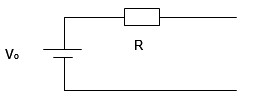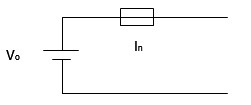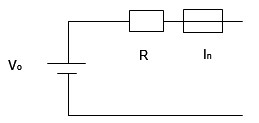When designing IS circuits, it it important to try to keep the intrinsic safety power levels in your circuit below the following thresholds depending on what you desire your maximum ambient temperature to be:

• 40°C: 1.3 Watts
• 50°C: 1.25 Watts
• 60°C: 1.2 Watts
• 70°C: 1.1 Watts
• 80°C: 1.0 Watts

When you stay below these limits, it helps simplify the intrinsic safety (IS) assessment by automatically allowing the following items from a maximum surface temperature standpoint as meeting T4 Temperature Classification without the need for further assessment or testing.

• All components that have a size greater than 20mm2
• All wiring
• All PCB traces

Power calculations for intrinsic safety are not based on the power a circuit uses during operation since intrinsic safety is based on normal and fault conditions.    Intrinsic safety power is calculated based on the maximum power that the intrinsic safety protective components will allow in the circuit.   In intrinsic safety circuits, power is typically limited by fuses, resistors, or a combination of fuses and resistors.

Below are some common circuits and the methods used to calculate the power.

Resistively Limited CircuitsPo = V2/4R

Where V = the maximum circuit voltage and R = the resistance of the protective resistor (with worst case tolerance)

Fuse Limited CircuitsPo = 1.7 * In * V

Where V = the maximum circuit voltage and In =the nominal rating of the fuse.

Combination of a Fuse and Resistor to Limit CircuitsWhen assessing these circuits, you must first determine if the fuse is actually contributing to the power  limitation.   This is done by comparing the fuse current (1.7 * In) to the current allowed by the resistor (Io = V/R).

If (1.7 * In) <  Io, then the fuse is helping to limit the power in the circuit and the following calculation is used:

Po = [V * (1.7 * In)] – [(1.7 * In)2 * R]

Where V = the maximum circuit voltage, In = the nominal rating of the fuse, and R= the resistance of the protective resistor (with worst case tolerance).

However, there is an additional exception where this formula cannot be used since the maximum power transfer occurs at a current of less than the maximum fuse current.

(1.7 *In) ≥ (V/(2*R))

In all other situations of fuse/resistor circuits where the formula above cannot be used, the following formula shall be used to calculate the power:

Po = V2/4R

Where V = the maximum circuit voltage and R = the resistance of the protective resistor (with worst case tolerance)